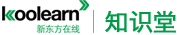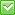<%/*%> <%*/%>热门：已解决问题
math

<div style="margin: 0in 0in 0pt"> 6-Q5
If b,c and d are constants and x^2+bx+c=(x+d)^2 for all values of x, what is the value of c?
(1) d=3
(2) b=6

stem(1):c=x(2d-b)+d^2 and d=3 we can not calculate the value of c (no sufficiency)
stem(2):b=6 same above (no sufficiency)
together (1) and (2): same above (no sufficiency)

please tell my why I am wrong.

6-Q16
<div style="margin: 0in 0in 0pt">Is I x-y I > I x-z I?
(1) IyI>IzI
(2)x<0

<div style="margin: 0in 0in 0pt">6-Q24
A certain restaurant offiers 6 kinds of cheese and 2 kinds of fruit for its dessert platter. If each dessert platter contains an equal number of kinds of cheese and kinds of fruit, how many different dessert platters could the restaurant offier?
A.8   B.12   C.15   D.21   E.27

6-Q26
K is a set of integers such that if the integer r is in K, then r+1 is also in K. Is 100 in K?
(1)50 is inK
(2)150 is in K

stem(1): 我们并不知道数列K的具体有多少个数， 所以不充分
stem(2): 同上
together (1) and (2): 50 is in K and 150 is in K, then 100 is in K (充分）

please tell me why I am wrong?

<div style="margin: 0in 0in 0pt">6-Q27
The sum of positive integers x and y is 77. What is the value of xy?
(1) x=y+1
(2)x and y have the same tens digit.

my question is  why stem(2) is sufficient condition?

6-Q33 <div style="margin: 0in 0in 0pt">A salesperson received a 6-percent commission on the amount of total sales up to and including \$10000, and an r-percent commission on the amount of total sales</div><div style="margin: 0in 0in 0pt">over \$10000. If the salesperson received a total commission of \$920 on total sales
of \$14000, what was the value of r?
A.3.2   B.4.3   C.6.6   D.8.0   E.9.2

6%*[10000+(14000-10000)] + r%*(14000-10000) = 920
r=2

please tell my why I am wrong?

<div style="margin: 0in 0in 0pt">6-Q35
In the xy-plane, what if the x-intercept of the line whose equation is 3y-4x=15?
A.-15/4   B.-4/3   C.4/3   D.15/4   E.5

y=(4/3)x + 5
</div></div></div></div></div></div>最佳答案

solve the second equation to get x^2+2dx+d^2

equating the result above to the first equation gives us

b = 2d
c = d^2

Note if you equate c = d^2 as in your analysis (2) then b has to be 2d for all values of x because for any other value of b it will be a different curves on the XY plane.

from A we know that d=3 so c = 9
from B we know that b=6=2d hence d = 3 and c = 9

6-Q16请复制题目，谢谢！

3：In each senario, the "cheese grid" will have six spaces and the "fruit grid" will have two spaces.

Scenario 1: "cheese grid" = YYNNNN; "fruit grid" = YY
Thus, the solution for the cheese is 6!/2!4! = 15 and the fruit is 2!/2! =1. Therefore, there are (15)(1) = 15 options

Scenario 2: "cheese grid" = YNNNNN; "fruit grid" = YN
Thus, the solution for the cheese is 6!/1!5! = 6 and the fruit is 2!/1!1! =2. Therefore, there are (6)(2) = 12 options

Scenario 1 + Scenario 2 = 27 Options. The correct answer is E.

4：K is a set of integers such that if the integer r is in K, then r + 1 is also in K

Statement 1:

50 is in K
that means 51 is in K. Similarly 52 is also in K.........on the same way 100 is also in K. Answer is YES
SUFF

Statement 2:
150 is in K.

This doesn't mean that 149 is in K. Only 151, 152...are in K.

Is 100 in K? answer is NO. SUFF

Hence D

5：X + Y = 77.....what is X & Y...?

Statement 1 ... X - Y = 1 ....based on this, we can calculate X & Y...Sufficient

Statement 2.... no proper equation can be derived....Insufficient

Hence A